Test: Information Theory & Coding

# Test: Information Theory & Coding - Electronics and Communication Engineering (ECE)

Test Description

## 10 Questions MCQ Test GATE ECE (Electronics) 2023 Mock Test Series - Test: Information Theory & Coding

Test: Information Theory & Coding for Electronics and Communication Engineering (ECE) 2023 is part of GATE ECE (Electronics) 2023 Mock Test Series preparation. The Test: Information Theory & Coding questions and answers have been prepared according to the Electronics and Communication Engineering (ECE) exam syllabus.The Test: Information Theory & Coding MCQs are made for Electronics and Communication Engineering (ECE) 2023 Exam. Find important definitions, questions, notes, meanings, examples, exercises, MCQs and online tests for Test: Information Theory & Coding below.
Solutions of Test: Information Theory & Coding questions in English are available as part of our GATE ECE (Electronics) 2023 Mock Test Series for Electronics and Communication Engineering (ECE) & Test: Information Theory & Coding solutions in Hindi for GATE ECE (Electronics) 2023 Mock Test Series course. Download more important topics, notes, lectures and mock test series for Electronics and Communication Engineering (ECE) Exam by signing up for free. Attempt Test: Information Theory & Coding | 10 questions in 30 minutes | Mock test for Electronics and Communication Engineering (ECE) preparation | Free important questions MCQ to study GATE ECE (Electronics) 2023 Mock Test Series for Electronics and Communication Engineering (ECE) Exam | Download free PDF with solutions
 1 Crore+ students have signed up on EduRev. Have you?
Test: Information Theory & Coding - Question 1

### The probabilities of the five possible outcomes of an experiment are given as: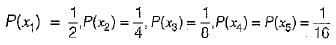If there are 16 outcomes per second then the rate of information would be equal to

Detailed Solution for Test: Information Theory & Coding - Question 1

The entropy of the system is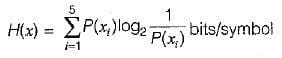or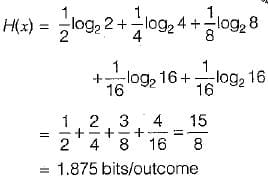Now, rate of outcome r= 16 outcomes/sec (Given)

∴ The rate of information R is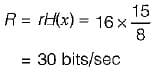Test: Information Theory & Coding - Question 2

### Consider the binary Hamming code of block length 31 and rate equal to (26/31). Its minimum distance is

Detailed Solution for Test: Information Theory & Coding - Question 2

Minimum distance in hamming code = 3.

Test: Information Theory & Coding - Question 3

### Assertion (A): The Shannon-Hartley law shows that we can exchange increased bandwidth for decreased signal power for a system with given capacity C. Reason (R): The bandwidth and the signal power place a restriction upon the rate of information that can be transmitted by a channel.

Detailed Solution for Test: Information Theory & Coding - Question 3

According to Shannon-Hartley faw, the channel capacity is expressed as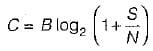Thus, if signal power is more, bandwidth will be less and vice-versa. Thus, assertion is a true statement. Reason is also a true statement because the rate of information that can be transmitted depends on bandwidth and signal to noise power. Thus, both assertion and reason are true but reason is not the correct explanation of assertion.

Test: Information Theory & Coding - Question 4

A 1200 band data stream is to be sent over a non-redundant frequency hopping system, The maximum bandwidth for the spread spectrum signal is 10 MHz. if no overlap occurs, the number of channels are equal to

Detailed Solution for Test: Information Theory & Coding - Question 4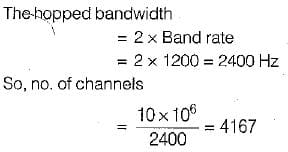Test: Information Theory & Coding - Question 5

The differential entropy H(x) of the uniformly distributed random variable* with the following probability density function for a = 1 is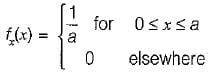Detailed Solution for Test: Information Theory & Coding - Question 5

We know that the differential entropy of x is given by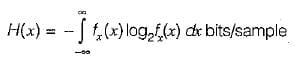Using the given probability density function, we have: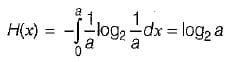for

a = 1, H(x) = log2 1 = 0

Test: Information Theory & Coding - Question 6

During transmission over a communication channel bit errors occurs independently with probability 1/2 . If a block of 3 bits are transmitted the probability of at least one bit error is equal to

Detailed Solution for Test: Information Theory & Coding - Question 6

(1 - p)n + np{ 1 - p)n-1 = Required probability
(Here, n = no. of bits and p = probability) ∴  Required probability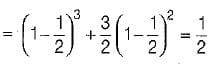Test: Information Theory & Coding - Question 7

A channel has a bandwidth of 8 kHz and signal to noise ratio of 31. For same channel capacity, if the signal to noise ratio is increased to 61, then, the new channel bandwidth would be equal to

Detailed Solution for Test: Information Theory & Coding - Question 7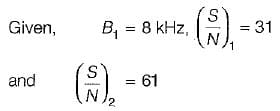We know that channel capacity is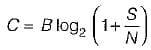Since channel capacity remains constant, therefore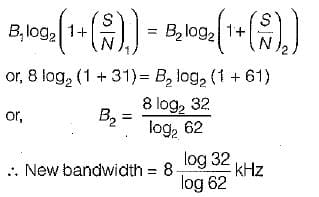Test: Information Theory & Coding - Question 8

A source generates 4 messages. The entropy of the source will be maximum when

Test: Information Theory & Coding - Question 9

A source delivers symbols m1, m2, m3 and m4 with probabilities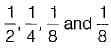respectively.

The entropy of the system is

Detailed Solution for Test: Information Theory & Coding - Question 9

The entropy of the system is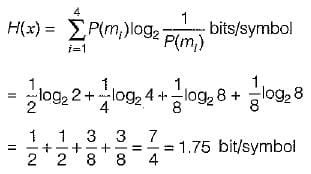Test: Information Theory & Coding - Question 10

A communication channel with AWGN has a BW of 4 kHz and an SNR pf 15. Its channel capacity is

Detailed Solution for Test: Information Theory & Coding - Question 10

Channel capacity is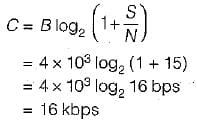## GATE ECE (Electronics) 2023 Mock Test Series

21 docs|263 tests
Information about Test: Information Theory & Coding Page
In this test you can find the Exam questions for Test: Information Theory & Coding solved & explained in the simplest way possible. Besides giving Questions and answers for Test: Information Theory & Coding, EduRev gives you an ample number of Online tests for practice

## GATE ECE (Electronics) 2023 Mock Test Series

21 docs|263 tests(Scan QR code)